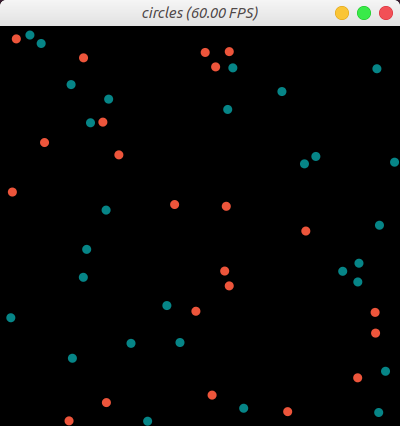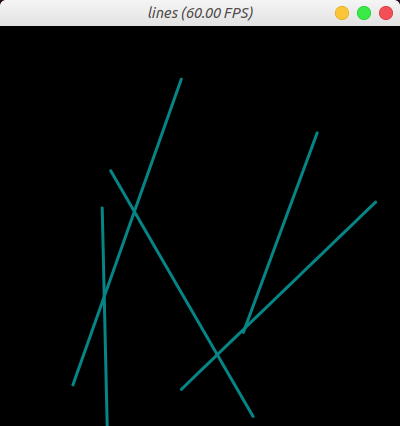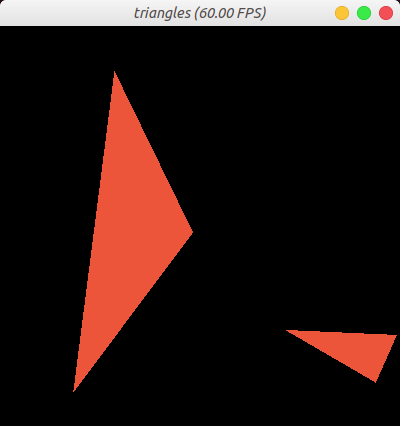Version: v1.5.0

# GUI System

Taichi has a built-in GUI system for visualizing simulation data in data containers like Taichi fields or NumPy ndarrays. It also has limited support for drawing primitive geometries.

## Create and display a window

The following code creates a `640x360` window with a "Hello World!" title, and displays it by calling `gui.show()`:

``gui = ti.GUI('Hello World!', (640, 360))while gui.running:    gui.show()``
##### note

Call `gui.show()` inside a `while` loop. Otherwise, the window would flash once and disappear.

## Close the window

You can set `gui.running=False` in the `while` loop to close the GUI:

``gui = ti.GUI('Window Title', (640, 360))while gui.running:    if some_events_happend:        gui.running = False    gui.show()``

## Coordinate system

Each window is built on a coordinate system: the origin is located in the lower-left corner, with the `+x` direction stretching to the right and the `+y` direction stretching upward.

## Display a field or ndarray

To display a Taichi field or a NumPy ndarray, call `gui.set_image()`. The method accepts both types as input.

``image = ti.Vector.field(3, ti.f32, shape=(640, 480))while gui.running:    gui.set_image(image)    gui.show()``

Because Taichi field is a global data container, if the vector field `image` is updated between the `while` loops, the GUI window refreshes to display the latest image.

##### IMPORTANT

Ensure that the shape of the input matches the resolution of the GUI window.

### Zero-copying frame buffer

In each loop of the `gui.set_image()` method call, the GUI system converts the image data to a displayable format and copies the result to the window buffer. This causes huge overload when the window size is large, making it hard to achieve high FPS (frames per second).

If you only need to call the `set_image()` method without using any drawing command, you can enable `fast_gui` mode for better performance. This mode allows Taichi GUI to write the image data directly to the frame buffer without additional copying, and significantly increases FPS.

``gui = ti.GUI(res, title, fast_gui=True)``

For this mode to work, ensure that the data passed into `gui.set_image()` is in a display-compatible format. In other words, If it is a Taichi field, ensure that it is one of the following:

• a vector field `ti.field(3, dtype, shape)` compatible with RGB format.
• a vector field `ti.field(4, dtype, shape)` compatible with RGBA format.

Note that `dtype` must be `ti.f32`, `ti.f64`, or `ti.u8`.

## Draw on a window

Taichi's GUI system supports drawing simple geometries, such as lines, triangles, rectangles, circles, and texts.

The `pos` parameter of every drawing method accepts Taichi fields or NumPy arrays, not Python primitive lists. Each element of the array is a pair of floats ranging from `0.0` to `1.0`, which represent the relative positions of the geometries. For example:

• `(0.0, 0.0)`: the lower-left corner of the window.
• `(1.0, 1.0)`: the upper-right corner of the window.

The following code draws 50 circles with a radius of `5` and in three different colors randomly assigned by `indices`, an integer array of the same size as `pos`.

``import numpy as nppos = np.random.random((50, 2))# Create an array of 50 integer elements whose values are randomly 0, 1, 2# 0 corresponds to 0x068587# 1 corresponds to 0xED553B# 2 corresponds to 0xEEEEF0indices = np.random.randint(0, 2, size=(50,))gui = ti.GUI("circles", res=(400, 400))while gui.running:    gui.circles(pos, radius=5, palette=[0x068587, 0xED553B, 0xEEEEF0], palette_indices=indices)    gui.show()``The following code draws five blue line segments whose width is 2, with `X` and `Y` representing the five starting points and the five ending points.

``import numpy as npX = np.random.random((5, 2))Y = np.random.random((5, 2))gui = ti.GUI("lines", res=(400, 400))while gui.running:    gui.lines(begin=X, end=Y, radius=2, color=0x068587)    gui.show()``The following code draws two orange triangles orange, with `X`, `Y`, and `Z` representing the three points of the triangles.

``import numpy as npX = np.random.random((2, 2))Y = np.random.random((2, 2))Z = np.random.random((2, 2))gui = ti.GUI("triangles", res=(400, 400))while gui.running:    gui.triangles(a=X, b=Y, c=Z, color=0xED553B)    gui.show()``## Event handling

Taichi's GUI system also provides a set of methods for mouse and keyboard control. Input events are classified into three types:

``ti.GUI.RELEASE  # key up or mouse button upti.GUI.PRESS    # key down or mouse button downti.GUI.MOTION   # mouse motion or mouse wheel``

Event key is the key that you press from your keyboard or mouse. It can be one of:

``# for ti.GUI.PRESS and ti.GUI.RELEASE event:ti.GUI.ESCAPE  # Escti.GUI.SHIFT   # Shiftti.GUI.LEFT    # Left Arrow'a'            # we use lowercase for alphabet'b'...ti.GUI.LMB     # Left Mouse Buttonti.GUI.RMB     # Right Mouse Button# for ti.GUI.MOTION event:ti.GUI.MOVE    # Mouse Movedti.GUI.WHEEL   # Mouse Wheel Scrolling``

An event filter is a combined list of key, type, and (type, key) tuple. For example:

``# if ESC pressed or released:gui.get_event(ti.GUI.ESCAPE)# if any key is pressed:gui.get_event(ti.GUI.PRESS)# if ESC is pressed or SPACE is released:gui.get_event((ti.GUI.PRESS, ti.GUI.ESCAPE), (ti.GUI.RELEASE, ti.GUI.SPACE))``

`gui.get_event()` pops an event from the queue and saves it to `gui.event`. For example:

``if gui.get_event():    print('Got event, key =', gui.event.key)``

The following code defines that the `while` loop goes on until ESC is pressed:

``gui = ti.GUI('Title', (640, 480))while not gui.get_event(ti.GUI.ESCAPE):    gui.set_image(img)    gui.show()``

`gui.is_pressed()` detects the pressed keys. As the following code snippet shows, you must use it together with `gui.get_event()`. Otherwise, it is not updated.

For example:

``while True:    gui.get_event()  # must be called before is_pressed    if gui.is_pressed('a', ti.GUI.LEFT):        print('Go left!')    elif gui.is_pressed('d', ti.GUI.RIGHT):        print('Go right!')``
##### caution

Call `gui.get_event()` before calling `gui.is_pressed()`. Otherwise, `gui.is_pressed()` does not take effect.

#### Retrieve cursor position

`gui.get_cursor_pos()` returns the cursor's current position in the window. The return value is a pair of floats in the range `[0.0, 1.0]`. For example:

``mouse_x, mouse_y = gui.get_cursor_pos()``

## GUI Widgets

Taichi's GUI system also provides widgets, including `slider()`, `label()`, and `button()`, for you to customize your control interface. Take a look at the following code snippet:

``import taichi as tigui = ti.GUI('GUI widgets')radius = gui.slider('Radius', 1, 50, step=1)xcoor = gui.label('X-coordinate')okay = gui.button('OK')xcoor.value = 0.5radius.value = 10while gui.running:    for e in gui.get_events(gui.PRESS):        if e.key == gui.ESCAPE:            gui.running = False        elif e.key == 'a':            xcoor.value -= 0.05        elif e.key == 'd':            xcoor.value += 0.05        elif e.key == 's':            radius.value -= 1        elif e.key == 'w':            radius.value += 1        elif e.key == okay:            print('OK clicked')    gui.circle((xcoor.value, 0.5), radius=radius.value)    gui.show()``